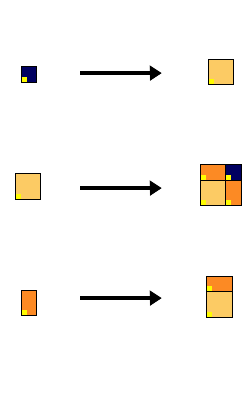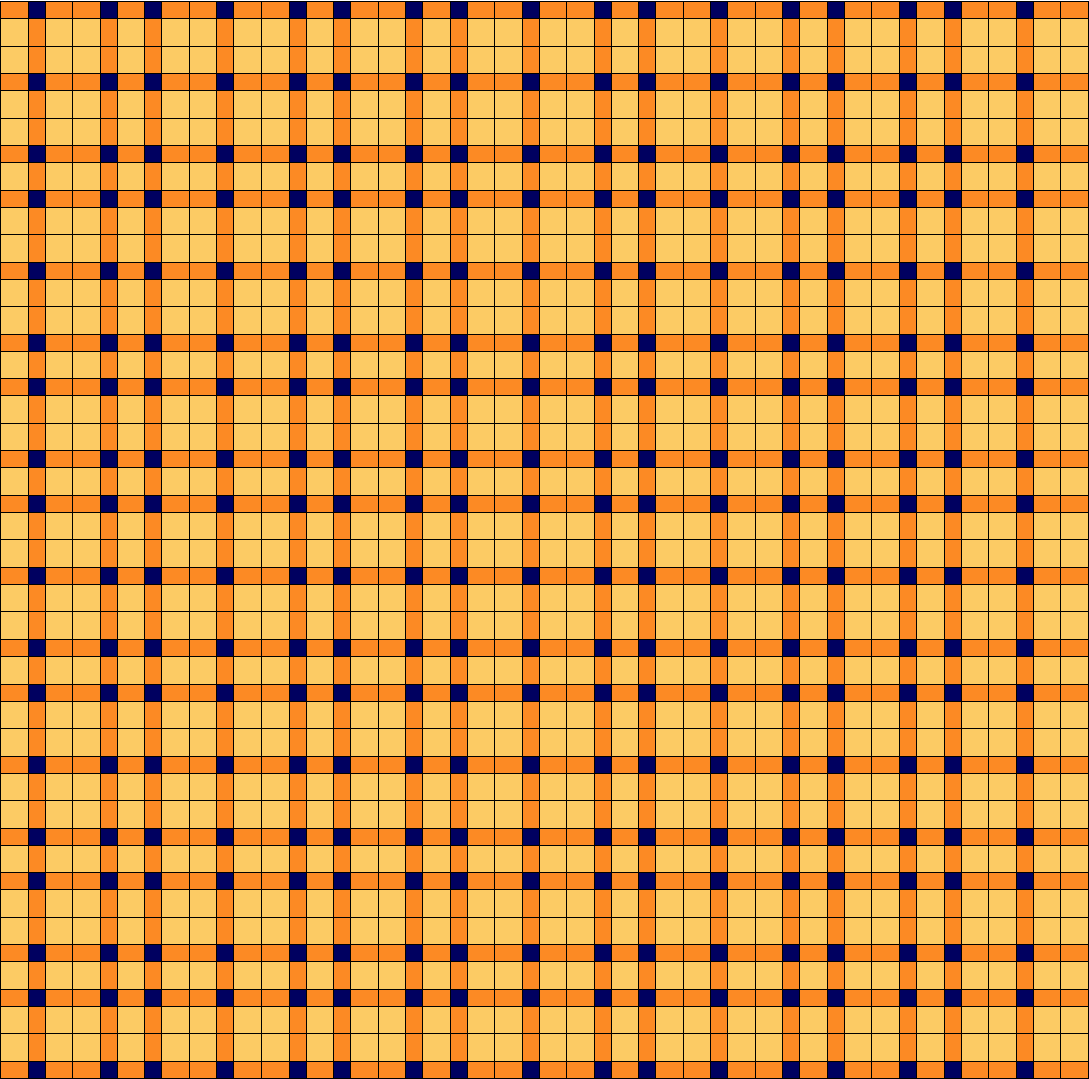## Fibonacci Times Fibonacci

### Info

The 2dim analogue of the famous Fibonacci tiling in one dimension. It is just the Cartesian product of two Fibonacci tilings $F_{1}$, $F_{2} : \{ T_{1} \times T_{2}\ |\ T_{i}\ in\ F_{i}\}$. Obviously, it can be generated by a substitution with three prototiles. It shares a lot of nice features with the 1dim Fibonacci tiling: It is a model set (better: it’s mld with one), so it has pure point spectrum. Its window is just a square. Moreover, it is its own dual tiling. Trivially, it allows a decoration with Ammann bars.

### Substitution Rule### Patch